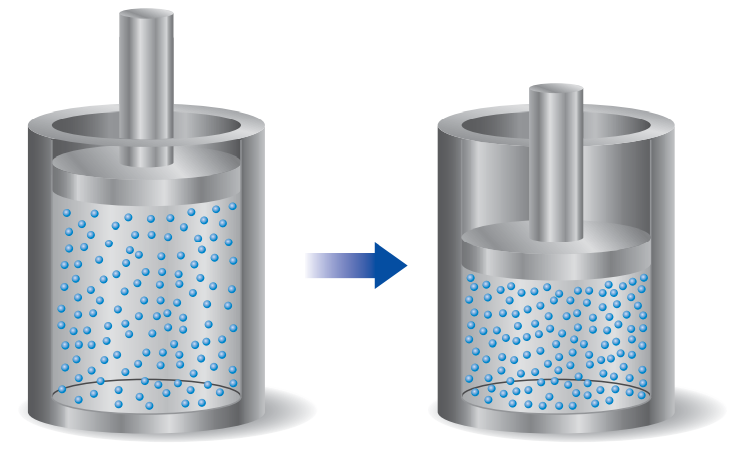×
Get Full Access to Chemistry: The Central Science - 12 Edition - Chapter 5 - Problem 96ae
Get Full Access to Chemistry: The Central Science - 12 Edition - Chapter 5 - Problem 96ae

×

# A sample of gas is contained in a cylinder-and-pistonISBN: 9780321696724 27

## Solution for problem 96AE Chapter 5

Chemistry: The Central Science | 12th Edition

• Textbook Solutions
• 2901 Step-by-step solutions solved by professors and subject experts
• Get 24/7 help from StudySoup virtual teaching assistantsChemistry: The Central Science | 12th Edition

4 5 1 379 Reviews
17
1
Problem 96AE

A sample of gas is contained in a cylinder-and-piston arrangement. It undergoes the change in state shown in the drawing. (a) Assume first that the cylinder and piston are perfect thermal insulators that do not allow heat to be transferred. What is the value of q for the state change? What is the sign of w for the state change? What can be said aboutE for the state change? (b) Now assume that the cylinder and piston are made up of a thermal conductor such as a metal. During the state change, the cylinder gets warmer to the touch. What is the sign of q for the state change in this case? Describe the difference in the state of the system at the end of the process in the two cases. What can you say about the relative values ofE?Step-by-Step Solution:
Step 1 of 3

Chapter 1: Sections 1-6 1.1 What is a Function A function is a rule that takes certain numbers as inputs and assigns to each a definite output number. Domain: the set of all input numbers Range: the set of all output numbers Independent Variable: input Dependent Variable: output Interval Notation: Closed Intervals: a ⦤ t ⦤b and is written [a,b] Open Intervals: a < t < b and is written (a,b) A mathematical model is a mathematical description of a real situation. Increasing and Decreasing Functions: A function is increasing if the values of f(x) increase as x increases A function is decreasing if the values of f(x) decrease as x increases 1.2 Linear Functions A linear function has a graph that has a straight line. Slope= ri

Step 2 of 3

Step 3 of 3

##### ISBN: 9780321696724

The answer to “?A sample of gas is contained in a cylinder-and-piston arrangement. It undergoes the change in state shown in the drawing. (a) Assume first that the cylinder and piston are perfect thermal insulators that do not allow heat to be transferred. What is the value of q for the state change? What is the sign of w for the state change? What can be said about E for the state change? (b) Now assume that the cylinder and piston are made up of a thermal conductor such as a metal. During the state change, the cylinder gets warmer to the touch. What is the sign of q for the state change in this case? Describe the difference in the state of the system at the end of the process in the two cases. What can you say about the relative values of E?” is broken down into a number of easy to follow steps, and 142 words. Since the solution to 96AE from 5 chapter was answered, more than 586 students have viewed the full step-by-step answer. This full solution covers the following key subjects: state, change, cylinder, piston, sign. This expansive textbook survival guide covers 49 chapters, and 5471 solutions. The full step-by-step solution to problem: 96AE from chapter: 5 was answered by , our top Chemistry solution expert on 04/03/17, 07:58AM. Chemistry: The Central Science was written by and is associated to the ISBN: 9780321696724. This textbook survival guide was created for the textbook: Chemistry: The Central Science, edition: 12.

Unlock Textbook Solution